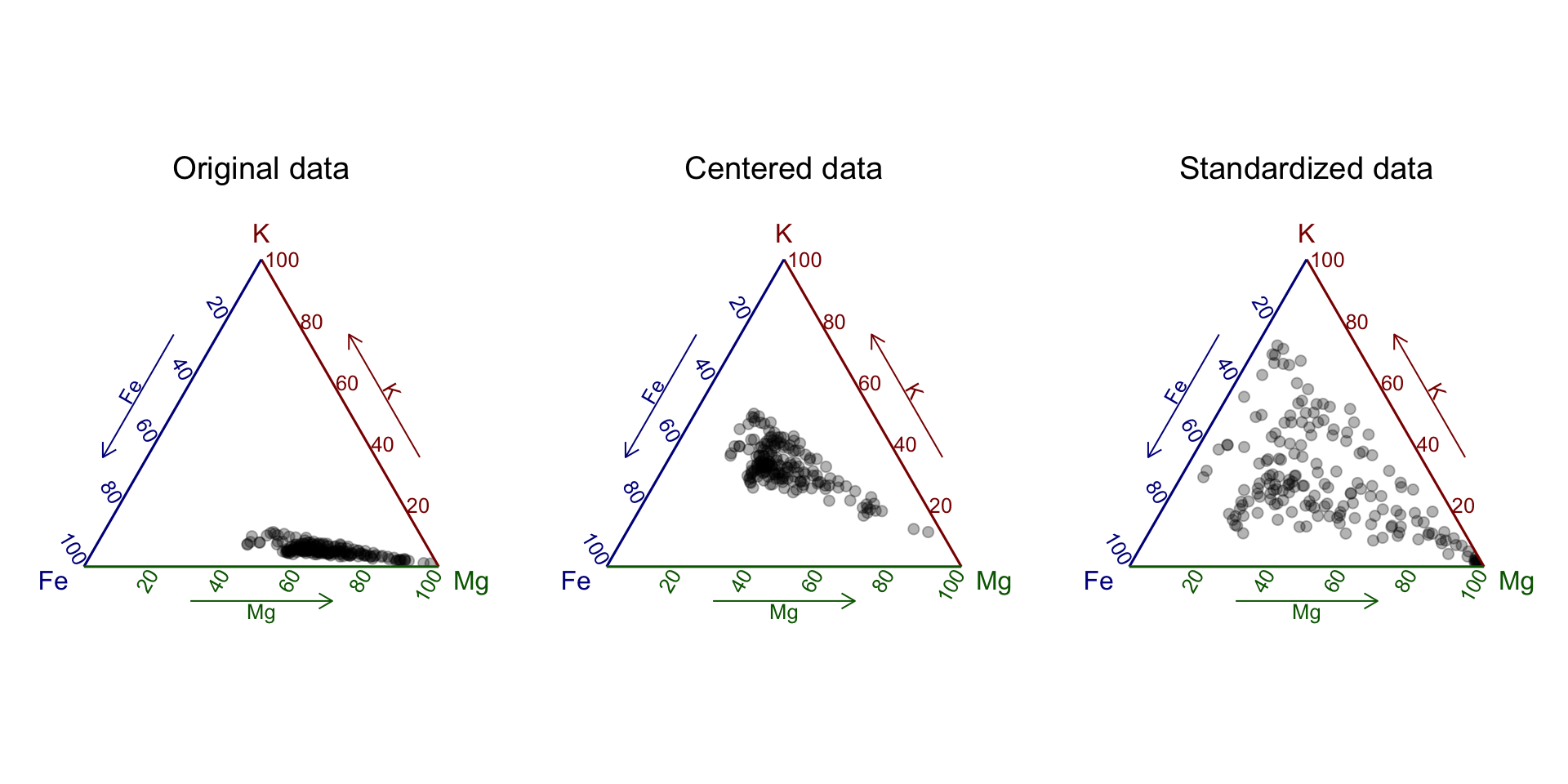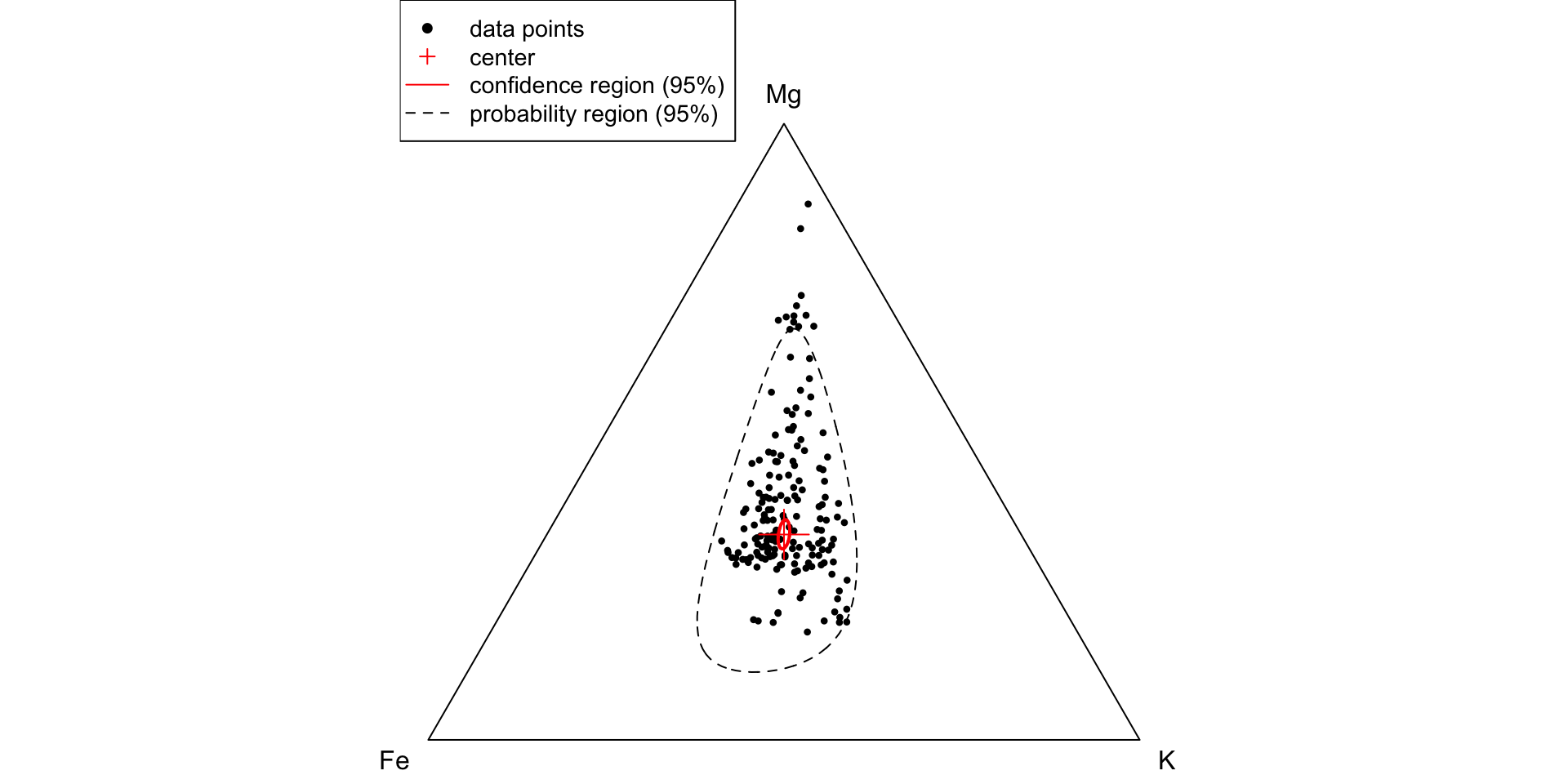Descriptive analysis of a compositional data set is based on the principle of working in coordinates and of working with log ratios. The most important functions for descriptive statistics in R are exemplified with a subcomposition of the data set of the geochemistry of a sediment core recovered from the eastern Juyanze palaeolake in north-western China (Hartmann and Wünnemann, 2009). We load the data set and prepare if for the analysis.

# load data set
sep='\t',
row.names = 'Sample')
# exclude columns of no interest
g36 <- g36[, -c(1:5, ncol(g36)-1, ncol(g36))]
# rename columns for better readability
colnames(g36) <- gsub(".mg.g", "", colnames(g36))

# define a subcomposition
library(compositions)
X = acomp(g36, c("Fe","K","Mg"))

#### Compositional mean

The center or compositional mean of a data set $$\mathbf X$$ with $$N$$ observations and $$D$$ parts is the composition

$\bar x = \frac{1}{N}\odot \bigoplus_{n=1}^N \mathbf x_n = \text{clr}^{-1} \left(\frac{1}{N} \sum_{n=1}^N \text{clr}(\mathbf x_n)\right) = \mathscr C \left[\exp \left(\frac{1}{N}\sum_{n=1}^N\ln(\mathbf x_n)\right)\right]\text{,}$

where $$x_n$$ is the nth observation, in the nth row of $$\mathbf X$$.

In R the compositional mean is computed by calling the mean() function on a acomp class object.

mean(X)
##           Fe            K           Mg
## "0.28247056" "0.05232328" "0.66520616"
## attr(,"class")
##  "acomp"

The compositional mean is not a robust estimator (van den Boogaart and Tolosana-Delgado 2013). The composition package provides a robust improvement of the compositional mean by computing the minimum covariance determinant (MCD) estimator. Therefore we have to add an additional argument robust = TRUE to the function call (mean(X, robust = TRUE)).

mean(X, robust = TRUE)
##           Fe            K           Mg
## "0.32229870" "0.05679286" "0.62090844"
## attr(,"class")
##  "acomp"

#### Metric variance and standard deviation

A global measure of spread is the metric variance, also known as total variance or generalized variance, which corresponds to the the average squared distance from the center of the data set (van den Boogaart and Tolosana-Delgado 2013).

$\text{mvar}(\mathbf X) = \frac{1}{N-1}\sum_{n=1}^N d^2_A(\mathbf x_n, \overline{\mathbf x})$

In R the computation of this variance type is provided by the compositions package by applying the mvar() function on a acomp class object:

mvar(X)
##  0.2507803

Van den Boogaart and Tolosana-Delgado 2013 define a metric standard deviation, to support quantitative interpretation:

$\text{msd}(X) = \sqrt{\frac{1}{D-1} \text{mvar}(X)}$

This quantity may be interpreted as the radial standard deviation on a log scale, if the variation was the same in all directions, or as some sort of average spread.

In R this equation is implemented in the msd() function of the compositions package.

msd(X)
##  0.3541047

#### Variation matrix

The variation of a composition among its components cannot be described by raw correlations or covariances. Instead Aitchison (1986) recommends the variation matrix. This matrix has $$D^2$$ components, each defined as

$\tau_{ij} = \text{var}\left(\ln\frac{x_i}{x_j}\right)$

and estimated by

$\tau_{ij}=\frac{1}{N-1}\sum_{n=1}^N\ln^2 \frac{x_{ni}}{x_{nj}} - \ln^2\frac{\bar x_i}{\bar x_j}\text{.}$

In R this variation matrix is provided by the compositions package by applying the variation() function on a acomp class object:

variation(X)
##            Fe          K        Mg
## Fe 0.00000000 0.06907321 0.3827192
## K  0.06907321 0.00000000 0.3005484
## Mg 0.38271916 0.30054839 0.0000000

The smaller a variation element is, the better the proportionality between the two components. To help in its interpretation, one may consider the transformation

$\rho_{ij} = \exp(-\tau_{ij}^2/2)$ and interpret it as a correlation coefficient (Van den Boogaart and Tolosana-Delgado 2013).

# interpreted like the correlation coefficient
exp(-variation(X)^2/2)
##           Fe         K        Mg
## Fe 1.0000000 0.9976173 0.9293806
## K  0.9976173 1.0000000 0.9558401
## Mg 0.9293806 0.9558401 1.0000000

#### Variance matrix

In order to analyse a compositional data set in a more classical way, as a matrix of covariances, the data set must be clr-transformed. The resulting clr-variance matrix is the variance-covariance matrix of the clr-transformed data set.

The covariance estimates $$\hat{\Sigma}_{ji}$$ are given by

$\hat{\Sigma}_{ji}=\text{cov}\left(\text{clr}_i(\mathbf x),\text{clr}_j(\mathbf x)\right) = \frac{1}{N}\sum_{n=1}^N \ln\frac{x_{ni}}{g(\mathbf x_n)}\cdot\ln\frac{x_{ni}}{g(\mathbf x_n)}-\ln\frac{\bar x_i}{g(\bar{\mathbf x})}\cdot\ln\frac{\bar x_j}{g(\bar{\mathbf x})}$ In R the covariance estimates may be computed by applying the var() function on a acomp class object.

var(X)
##             Fe           K          Mg
## Fe  0.06700404  0.01877231 -0.08577634
## K   0.01877231  0.03961378 -0.05838609
## Mg -0.08577634 -0.05838609  0.14416243

#### Standardization, centering, scaling

In many statistical analysis it is useful to center the data by removing the mean. In compositional data analysis, we can achieve this task by perturbing the composition by the inverse of its center:

$\mathbf X^* = \mathbf X \ominus \bar{\mathbf x}$

Similarly, we may scale (standardize) a compositional data set, by using perturbation and powering. A data set is centered by perturbation with the inverse of the center and scaled by powering with the inverse of the square root of the metric variance:

$\mathbf Z = \frac{1}{\sqrt{\text{mvar}(\mathbf X)}} \odot (\mathbf X \ominus \bar{\mathbf x})$

In R we may explicitly write (X - mean(X)) / sqrt(mvar(X)) or we make use of the handy scale() function provided by the compositions package.

library(ggtern)

grobs = list()
data = list(X,
X-mean(X),
scale(X,center=TRUE,scale=TRUE))
titles <-  c('Original data',
'Centered data',
'Standardized data')

for (p in 1:3){
grobs[[p]] <- ggtern(data = as.data.frame(data[[p]]), aes(Fe, K, Mg)) +
geom_point(alpha = 0.3, size = 2, color = "black") +
theme_rgbw() + ggtitle(titles[p]) +
theme(plot.title = element_text(hjust = 0.5))
}
ggtern::grid.arrange(grobs=grobs, nrow=1)#### Normal-based predictive and confidence regions

In order to represent the center and dispersion structure of compositional data graphically, we may draw two ellipses. One corresponds to a p-confidence region of the mean. This confidence region is defined by its radius r according to a Fisher $$F-$$distribution of $$(D - 1)$$ and $$N-D+1$$ degrees of freedom:

$r = \sqrt{\frac{D-1}{N-D+1}\cdot F_p(D-1, N-D+1)}$

This is valid if the data follows a normal distribution for compositions or else if the number of observations $$N$$ is large. In this last case, a compositional central limit theorem applies.

The other ellipsis corresponds to a p-probability region, defined by its radius given by the $$\chi^2$$ distribution of $$(D-1)$$ degrees of freedom:

$r = \sqrt{\chi^2_{\alpha}(D-1)}$

We show this graphical procedure on a subcomposition of the data set of the geochemistry of a sediment core recovered from the eastern Juyanze palaeolake in north-western China (Hartmann and Wünnemann, 2009). We load the data set and prepare if for the analysis.

### DATA PREPARATION ###
sep='\t',
row.names = 'Sample')
# exclude columns of no interest
g36 <- g36[, -c(1:5, ncol(g36)-1, ncol(g36))]
# rename columns for better readability
colnames(g36) <- gsub(".mg.g", "", colnames(g36))
# define a subcomposition
X = acomp(g36, c("Fe","K","Mg"))

### COMPUTATION OF MEAN, VARIANCE AND RADII ###
# center the subcomposition
X = X - mean(X, robust = TRUE)
# get the mean:
mn = mean(X, robust = TRUE)
vr = var(X)
df1 = ncol(X)-1 # degree of freedom
df2 = nrow(X)-ncol(X)+1 # degree of freedom
rconf = sqrt(qf(p = 0.95, df1, df2)*df1/df2 )
rprob = sqrt(qchisq(p = 0.95, df = df1))

### PLOTTING ###
par(mar=c(0.5,0,0,0))
# plot data points:
plot.acomp(X, pch=16, cex = 0.6) # data points
plot.acomp(mn, pch = 3, cex = 3, col = 'red',  add = TRUE) # center
# plot the ellipses:
ellipses(mean = mn, var = vr, r = rconf, col = "red", lwd = 2) # confidence region for the mean
ellipses(mean = mn, var = vr, r = rprob, lty = 2) # probability region for the data
legend('topleft',
legend = c('data points',
'center',
'confidence region (95%)',
'probability region (95%)'),
pch = c(16,3,NA,NA),
lty = c(NA,NA,1,2),
col = c('black','red','red','black'),
cex = 0.9)#### The summary() function for a acomp object

As for many other object classes of R there exists as well a summary() function for a acomp object. The summary() function returns exhaustive amount of information, including descriptive statistics, for a particular acomp class object. Refer to the documentation for detailed information on the function output (help(summary.acomp))

summary(X)
## $mean ## Fe K Mg ## "0.3054736" "0.3211148" "0.3734116" ## attr(,"class") ##  "acomp" ## ##$mean.ratio
##          Fe         K        Mg
## Fe 1.000000 0.9512909 0.8180615
## K  1.051203 1.0000000 0.8599488
## Mg 1.222402 1.1628600 1.0000000
##
## $variation ## Fe K Mg ## Fe 0.00000000 0.06907321 0.3827192 ## K 0.06907321 0.00000000 0.3005484 ## Mg 0.38271916 0.30054839 0.0000000 ## ##$expsd
##          Fe        K       Mg
## Fe 1.000000 1.300590 1.856407
## K  1.300590 1.000000 1.730176
## Mg 1.856407 1.730176 1.000000
##
## $invexpsd ## Fe K Mg ## Fe 1.0000000 0.768882 0.5386749 ## K 0.7688820 1.000000 0.5779760 ## Mg 0.5386749 0.577976 1.0000000 ## ##$min
##           Fe         K         Mg
## Fe 1.0000000 0.3144301 0.03582797
## K  0.5887497 1.0000000 0.11394575
## Mg 0.4380004 0.3883320 1.00000000
##
## $q1 ## Fe K Mg ## Fe 1.0000000 0.7783108 0.6486084 ## K 0.8677156 1.0000000 0.6785914 ## Mg 0.8357302 0.8550891 1.0000000 ## ##$med
##         Fe         K        Mg
## Fe 1.00000 0.9683262 0.9604579
## K  1.03271 1.0000000 0.9420606
## Mg 1.04117 1.0615028 1.0000000
##
## $q3 ## Fe K Mg ## Fe 1.000000 1.152451 1.196558 ## K 1.284834 1.000000 1.169469 ## Mg 1.541762 1.473641 1.000000 ## ##$max
##           Fe        K       Mg
## Fe  1.000000 1.698515 2.283103
## K   3.180357 1.000000 2.575116
## Mg 27.911152 8.776106 1.000000
##
## \$missingness
##         missingType
## variable NMV BDL MAR MNAR  SZ Err
##       Fe 177   0   0    0   0   0
##       K  177   0   0    0   0   0
##       Mg 177   0   0    0   0   0
##
## attr(,"class")
##  "summary.acomp"

Citation

The E-Learning project SOGA-R was developed at the Department of Earth Sciences by Kai Hartmann, Joachim Krois and Annette Rudolph. You can reach us via mail by soga[at]zedat.fu-berlin.de.You may use this project freely under the Creative Commons Attribution-ShareAlike 4.0 International License.

Please cite as follow: Hartmann, K., Krois, J., Rudolph, A. (2023): Statistics and Geodata Analysis using R (SOGA-R). Department of Earth Sciences, Freie Universitaet Berlin.